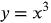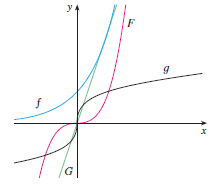1
113
views
4c
Problem

Problem 4c

Chapter 1: Functions and ModelsTextbook ExpertVerified Tutor
18 Oct 2021

Given information

The given is:

To match the equationwith its graphs without using a computer or graphing calculator.Step-by-step explanation

Step 1.

Explanation:

The equationrepresents a polynomial function that is cubic in nature.